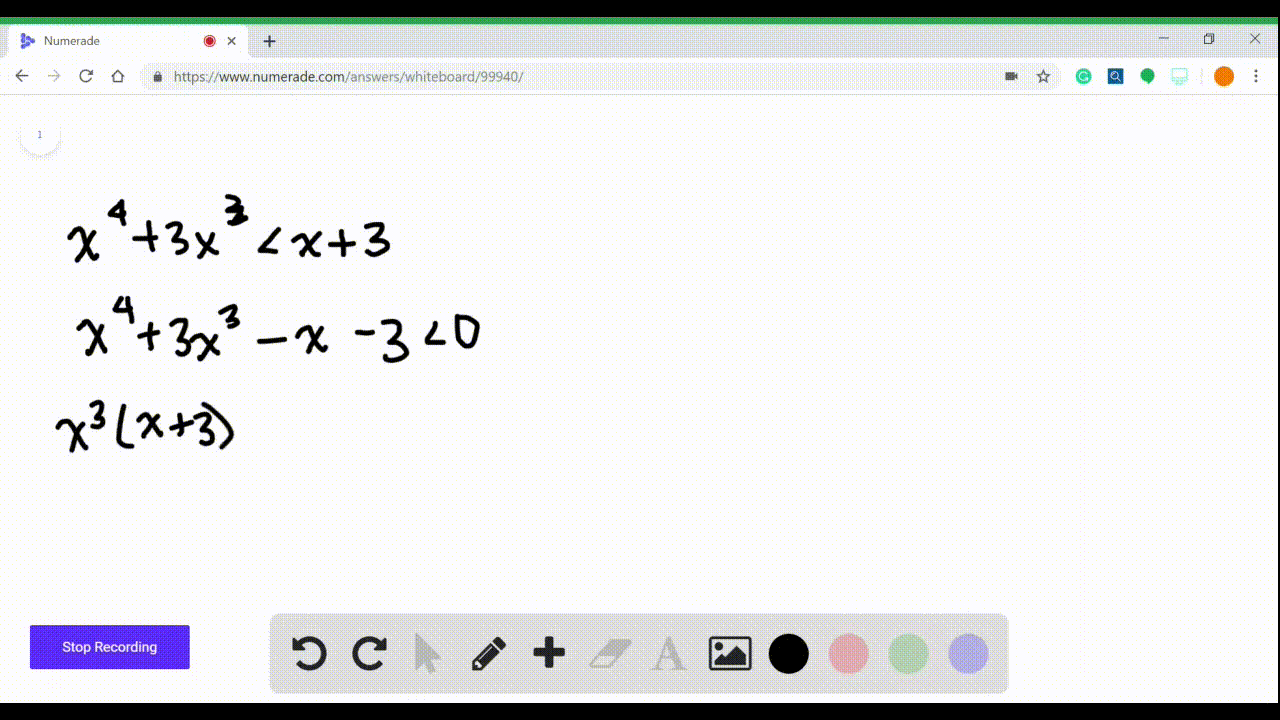Enroll in one of our FREE online STEM summer camps. Space is limited so join now!View Summer Courses### $3-16=$ Polynomial Inequalities Solve the inequal…

03:47Other Schools

Need more help? Fill out this quick form to get professional live tutoring.

Get live tutoring
Problem 9

$3-16=$ Polynomial Inequalities Solve the inequality.
$$2 x^{3}-x^{2}<9-18 x$$

## Discussion

You must be signed in to discuss.

## Video Transcript

the question asked us to solve this inequalities. We have two x cubed minus X squared, and that is less the nine minus 18 X. So what I'm gonna do first is take everything to one side. So to x cubed minus X squared and then plus 18 x minus nine, it is less than zero. They're gonna try to factor. So I got my acts on the 18. So I'm gonna factor by grouping and benefactor, these two. I'm gonna take out an X squared and I'm gonna be left with two X minus one. And it needs to I'm gonna take out a common factor of nine, and I'm gonna be laughing, too. X minus one is less than zero. So that gives me X squared. Plus nine multiplied by two X minus. One is less than zero. So that's how it factors. This doesn't factor any further. So we actually get X squared is equal to minus nine. We say that's not possible. So there's no solution there because it would mean that something squared is negative and that just does not happen. And the other option is that two x minus one is equal to zero which means that two X is equal to one, which means X is 1/2. So our only real concern is the 1/2. So we're gonna have a very simple number line where we just have 1/2 and I just need to know what the number before 1/2. So let's plug in zero. Let's say what happens when x zero and all we're looking at is the X squared plus nine and the two X minus one. And then the combination of that. Okay, so you don't have to necessarily write this out every time. I'm just gonna show you what we do. So when I put in zero, I get positive. Nine zero minus one is negative, Which means this is negative. So before that, 1/2 its negative and then you need something bigger than that. So I'm gonna say when X is one, I get one squared plus nines. That's still positive. Uh, two times one minus one is positive one. So this is positive. So it's positive after, So I want to know what it's less than zero. So my solution is the negative part. So my solution is the interval from minus infinity up to 1/2 because that is where it's negative